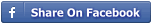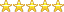Home»Economics» The determinants of balance of payment in Nigeria

# The determinants of balance of payment in Nigeria

Department:Economics
By:mattkingsRating:(5.0) votes1
Rate this project
Price:₦1000
Abstract
This study aimed at analyzing through econometric methodology the Determinant of Balance of payment in Nigeria. In the work, we capture balance of payment as the dependent variable while trade openness, external debt service and exchange rate as the explanatory variable. In the second page of the regression estimated we observed GDP as the dependent variable while balance of payment, trade openness exchange rate and external debt service being the independent variable. An ordinary least square was used to capture the relationship between the variables been the regression plane. From the result estimated, we observed that all parameters are statistically significant from the t-test statistics. We also realize from the F-test estimation that the model is statistically insignificant because the T-cal  T-tab in all the variable. The result went further in the test of autocorrelation through the Durbin-Watson that there is absence of autocorrelation among the variable. ...
Preview +
Other Economics project topics and materials you might be interested in